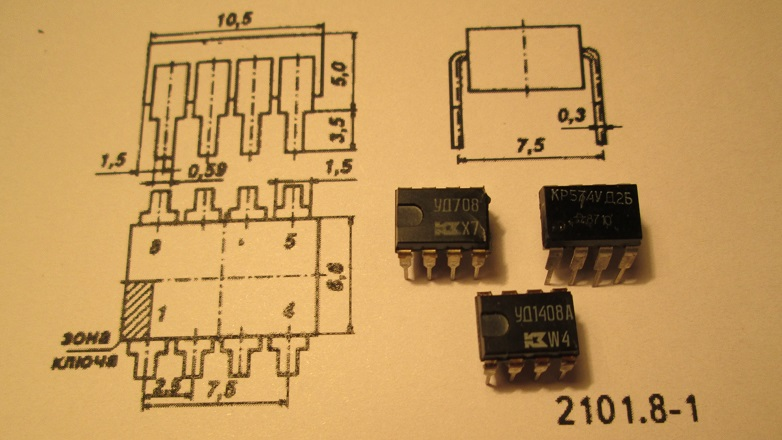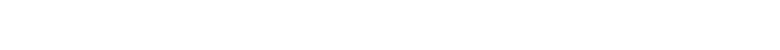# Calculation of the sum, difference, integral and derivative on the op-ampIn the previous publication of the cycle, we figured out how to calculate a proportional (amplifying) link on a real operational amplifier, taking into account its static and dynamic characteristics.

In this publication of the cycle, we will learn how to use the op-amp to perform addition and subtraction operations. In addition, we will analyze the work of integrating and differentiating links, as well as sample-and-hold schemes.

At KDPV, to the company of operational amplifiers K140UD708 and K574UD2B, a precision op-amp K140UD1408, the Soviet analogue of LM308, was added.

For those who joined recently, I inform you that this is the third of six publications in the cycle. The content of publications with links to them is at the end of the article.

## summing amplifier

The addition operation on the op-amp can be performed using a summing amplifier. The simplest summing amplifier can be obtained by adding a resistor to the op-amp inverting amplifier circuit:Further, similarly to the method for calculating the transfer characteristic of an inverting amplifier. There is a potential of 0 V at the inverting input of the op-amp. The sum of the input currents through the input resistors, due to the presence of voltages U at the inputs

in1

and U

vx2

, is compensated by the current through the feedback resistor R2. The voltage drops across the input resistors are numerically equal to U

in1

and U

vx2

the voltage drop across the resistor R2 is equal to U

exit

.

The transfer characteristic of the simplest summing amplifier with equal resistances of the input resistors can be represented as a formula:

The resistance of the resistor R3 connected to the non-inverting input of the op-amp to compensate for the bias current is equal to the resistance of the resistors in the OOS circuit connected in parallel.

For the correct operation of the summing link, the signal sources must have the lowest possible output impedance so that the result of the calculations is not affected by the low input resistance of the link, and the signal sources do not shunt each other.

## Difference amplifier

The subtraction operation on the op-amp can be performed using a difference amplifier. The circuit of the difference (differential) amplifier can also be obtained by refining the circuit of the inverting amplifier on the op-amp:For signal U

in1

the circuit behaves like a non-inverting amplifier, and for the signal U

vx2

– as inverting. The transfer characteristic of the simplest difference amplifier, under the condition of pairwise equality of resistances R1 = R3 and R2 = R4, can be expressed by the formula:

The circuit of the simplest difference amplifier is simple and clear, but does not reflect the complexity of the behavior of this link:

1. The inputs of the simplest difference amplifier at R1 = R3 and R2 = R4, however, have different input impedance, i.e. affect input sources differently.
2. The output impedance of the simplest difference amplifier varies as a result of calculations, depending on which amplifier (inverting or non-inverting) it is in this particular case.
3. When changing the transmission coefficient of the simplest difference amplifier, careful selection of the values ​​of all four resistors is required to ensure both the approximate equality of the input resistances and the required transmission coefficients for each input.

## Instrumentation amplifier

The measuring (instrumental) amplifier is a difference amplifier with the same input resistance and the ability to adjust the transfer coefficient by changing the value of only one resistor in the OOS circuit:Compared with the simplest difference amplifier, the circuit is much more complicated, but the adjustment of the transfer coefficient is reduced to the selection of the resistance of only one resistor R1. The rest of the circuit can be placed on a single chip, which improves manufacturability and makes it easier to ensure the equality of the resistances of the remaining resistors.

The gain of the measuring amplifier is calculated by the formula:

The Instrumentation Amplifier has a number of remarkable features:

1. If R1 is excluded from the circuit (R1 = ∞), the transmission coefficient of the measuring amplifier becomes equal to unity, and the output voltage will be equal to the voltage difference at the inputs.
2. If voltage U is applied to the upper input of the measuring amplifierin1 = 0 V, it can be used as an inverting amplifier with high input impedance.
3. If voltage U is applied to the lower input of the measuring amplifiervx2 = 0 V, it can be used as a “classic” non-inverting amplifier.
4. The high input impedance allows you to connect a summing resistor to each input, as in a summing amplifier circuit.

The above features make it possible to use the scheme as a universal tool. Measuring amplifiers are produced ready-made by the industry. A limiting factor in the use of instrumentation amplifiers is their increased cost, so they are usually used in critical high-budget solutions.

The integrator is designed to calculate the integral over time. The link is inertial:The transfer coefficient of the integrating link at R2 = ∞:

Integrators are usually designed around FET op amps with negligible input currents to minimize output voltage drift. If it is not possible to get rid of the drift, a resistor R2 with a resistance of the order of units or tens of MΩ is connected in parallel with the capacitor to ensure direct current OOS.

Resistor R2 degrades the integrating properties of the link at very low frequencies and reduces stability. If it is not possible to get rid of the direct current feedback in the integrating link, it makes sense to try to replace R2 with an equivalent T-bridge using the method given in the first publication of the cycle.

The differentiating link is designed to calculate the time derivative:Transfer coefficient of the differentiating link at C2 = 0:

Differentiating links are also developed on the basis of an op-amp with field-effect transistors at the input and negligibly small input currents. Capacitor C2 serves to reduce the sensitivity of the link to high-frequency interference and increase the stability of the link at the upper frequencies of the operating range.

## Sample-hold scheme

Sample-hold circuits are used to record and store the value of an analog signal:When the contacts S2 are closed, the value written to the capacitor C1 is reset. When the contacts S1 are closed, the value U is written to C1

in

. When S1 opens, the written value is stored in C1.

As DA2, an op-amp with field-effect transistors at the input should be used. Capacitors with low leakage current should be used as C1.

## PID controller

All links considered within the framework of the publication are united by the fact that they are used in PID controllers:PID controller is a device that generates a control action

u

Mismatch signal generation scheme

e

The differential amplifier appears in the text as “difference” so as not to be confused with the differentiating link.

The issues of estimating the calculation error remained outside the scope of the publication, but if necessary, all the techniques necessary for this can be found in .

From the next publication of the cycle, we will learn: how to implement the multiplication function on the op-amp, how to compare two signals by value, how to find the largest value, and much more.

This series of publications consists of six parts. Summary of publications:

1. Prerequisites for the emergence of OS. “Ideal” operational amplifier. Inverting and non-inverting amplifiers, repeater.
2. Differences between a “real” OS and an “ideal” one. The main characteristics of a real OS. Restrictions of real OS.
3. Summing amplifier. difference amplifier. measuring amplifier. integrating link. differentiating link. Sample-hold scheme. < — Are you here
4. Active detector. Active peak detector. logarithmic amplifier. Active signal limiter. Op-amp comparator. Reference voltage source. Current source. Amplifier.
5. Frequency-dependent feedback in the OS. Active filters on the OS. Signal generators on the OS.
6. Unipolar inclusion of the OS. Input interference, “decoupling” and protection of input circuits, shielding.

### ▍ Sources used:

1. Gutnikov. Integrated electronics in measuring devices. Energoatomizdat, 1988

2. Horowitz, Hill. The art of circuitry. 2nd ed. Peace, 1993

3. Tietze, Shenk. Semiconductor circuitry. 5th ed. Peace, 1982

4. Shkritek. A reference guide to audio circuitry. Peace, 1991